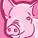# QlikView App Dev

Discussion Board for collaboration related to QlikView App Development.

Announcements
Join this live chat April 6, 10AM EST - QlikView to Qlik Sense REGISTER
cancel
Showing results for
Search instead for
Did you mean:Contributor

## Calculate percentage

Hi,

I have an excel table attached to this question.

Here is wat i want to achieve:

We want to calculatie (based on any selection) the total percentage of In Full. So depending on if the artikel is a piece (aantal) or KG. it should calculate de deliveryperformance.

The outcome of this set of data should be a KPI on total: 102,73%.

The files in Qlik sense are dimension and meassurements:

Dimensions are:

Datum, Deb.Nr, Deb.Naam, Art.Nr, Art.Naam, Aantal, Gewicht, RegAantal, NettoKg, Tijd.

Meassurements are:

Stuksartikel : IF(Stuksartikel = 'Ja','Ja','Nee')

#/KG Besteld: IF(Stuksartikel = 'Ja', Sum(Aantal), Sum(Gewicht))

#/KG Geleverd: IF(Stuksartikel = 'Ja', Sum(RegAantal),Sum([NettoKg]))

% #/KG Uitgeleverd: SUM(Distinct IF(Stuksartikel = 'Ja', RegAantal,NettoKg))/SUM(Distinct IF(Stuksartikel = 'Ja', Aantal,Gewicht))

Hope anyone can give me some idea’s how to make this KPI.

5 RepliesPartner

Hi,

Based on the calculation of your excel, I think the KPI expression should be :

```=(
Sum({<Stuksartikel = {"Ja"}>}RegAantal) +
Sum({<Stuksartikel = {"Nee"}>}NettoKg)
)
/
(
Sum({<Stuksartikel = {"Ja"}>}Aantal) +
Sum({<Stuksartikel = {"Nee"}>}Gewicht)
)```

Please try.

AiolosContributor
Author
Hi Aiolos, With this formula, the outcome is 97,47%. It should be 102,73%Partner

I don't know whether you can open my attachment, but it works in my desktop:If you still can't get the correct answer, could you please share your example?

it's fine for me to open qlikview or qliksense, then I can check why it's not correct.

Thanks.

Aiolos ZhaoContributor
Author

Hi Aiolos,

thanks for the solution. I had to change something in my app, now it works with your Formula. My only problem now is that when i select only the stuksartikel or only the kg artikel the outcome stays the same.

Do you have any idea?Partner
Hi,
I don't see the column named [kg artikel],
but about the stuksartikel , yes, the result won't change.
because I hard-code the logic about the stuksartikel in the expression, like what you see:
Stuksartikel = {"Ja"}
Stuksartikel = {"Nee"}
Because I followed the logic in your excel, so if you think the logic is wrong, could you please give me a new logic to calculate what you want, then I will try to find a new expression to solve that.
Aiolos Zhao